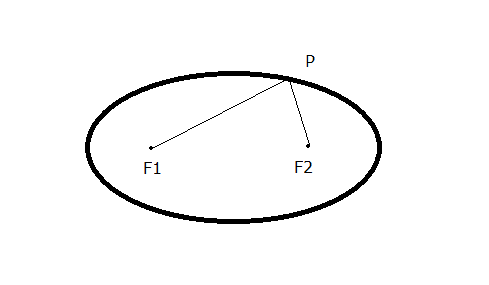# What are the foci of an ellipse?

• B
• avito009

#### avito009

What is a foci of an ellipse?

The foci, an ellipse is the locus of points such that the sum of the distance to each focus is constant.

Can someone explain this in simple terms?

One way to look at it is how you might draw an ellipse using a pencil, paper, two thumb tacks and a loop of string.

Placing the thumb tacks in the middle of the paper some small distance apart, say 2 inches and then wrapping the string around both thumb tacks and the pencil to create a triangle. Now move the pencil clockwise around keeping the string taut to create an ellipse. From this you can see that the two thumb tacks control the shape of the ellipse. They are in fact at the foci of the ellipse.

Here's another related way to draw it:

http://www.mathopenref.com/printellipse1.html

•mfb and Nidum
So you mean that suppose I take the same thumb tacks but I pull the string with a bit lesser force and draw the ellipse. Now I pull the string till as much as it can stretch and then draw an ellipse. Now in the first case if the sum of distances of all the points is 12. In the latter case when i pull the string hard also the sum of all the distances will be 12. Am I right? But for a point that is not the focus the sum of distances will vary when the shape changes. Am I right?

So you mean that suppose I take the same thumb tacks but I pull the string with a bit lesser force. Now I pull the string till as much as it can stretch. Now in the first case if the sum of distances of all the points is 12. In the latter case when i pull the string hard also the sum of all the distances will be 12. Am I right? But for a point that is not the focus the sum of distances will vary when the shape changes. Am I right?
The idea with the string is that you pull it so that it is taut.
Here is a picture of what jedishrfu is talking about. The foci (plural of focus) are at F1and F2 and P is an arbitrary point on the ellipse. For an ellipse, the sum of the lengths of the segments F1P and PF2 is a constant. As we move around the ellipse to the right, the segment F1P gets longer and the segement PF2 gets shorter, but the sum F1P + PF2 remains the same.

If you move one or both of the thumbtacks, which changes the location of the foci, you get a different ellipse.What is a foci of an ellipse?

The foci, an ellipse is the locus of points such that the sum of the distance to each focus is constant.
Your sentence above is very confused, as it implies that "the foci" is the locus of some points. That's not true.

You hold the string taut and the string does not stretch then the pencil will draw the ellipse correctly. Notice at each point on the ellipse, the string makes a triangle with corners being the pencil point and the two thumb tacks, and for each of these triangles the triangle perimeter is always the same length.

The idea with the string is that you pull it so that it is taut.
Here is a picture of what jedishrfu is talking about. The foci (plural of focus) are at F1and F2 and P is an arbitrary point on the ellipse. For an ellipse, the sum of the lengths of the segments F1P and PF2 is a constant. As we move around the ellipse to the right, the segment F1P gets longer and the segement PF2 gets shorter, but the sum F1P + PF2 remains the same.

If you move one or both of the thumbtacks, which changes the location of the foci, you get a different ellipse.

Thanks a lot. That is as simple as it can get. I get the point.